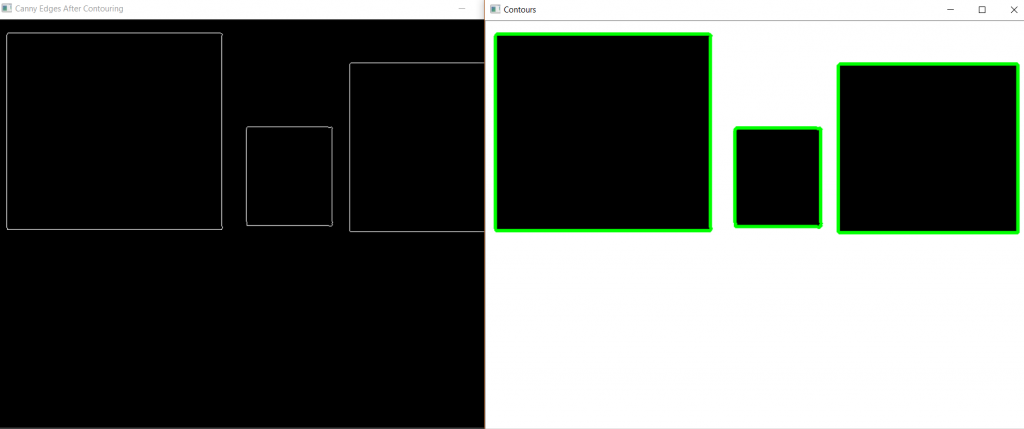# Find and Draw Contours using OpenCV | Python

Contours are defined as the line joining all the points along the boundary of an image that are having the same intensity. Contours come handy in shape analysis, finding the size of the object of interest, and object detection.

OpenCV has `findContour()` function that helps in extracting the contours from the image. It works best on binary images, so we should first apply thresholding techniques, Sobel edges, etc.

Below is the code for finding contours –

 `import` `cv2 ` `import` `numpy as np ` ` `  `# Let's load a simple image with 3 black squares ` `image ``=` `cv2.imread(``'C://Users//gfg//shapes.jpg'``) ` `cv2.waitKey(``0``) ` ` `  `# Grayscale ` `gray ``=` `cv2.cvtColor(image, cv2.COLOR_BGR2GRAY) ` ` `  `# Find Canny edges ` `edged ``=` `cv2.Canny(gray, ``30``, ``200``) ` `cv2.waitKey(``0``) ` ` `  `# Finding Contours ` `# Use a copy of the image e.g. edged.copy() ` `# since findContours alters the image ` `contours, hierarchy ``=` `cv2.findContours(edged,  ` `    ``cv2.RETR_EXTERNAL, cv2.CHAIN_APPROX_NONE) ` ` `  `cv2.imshow(``'Canny Edges After Contouring'``, edged) ` `cv2.waitKey(``0``) ` ` `  `print``(``"Number of Contours found = "` `+` `str``(``len``(contours))) ` ` `  `# Draw all contours ` `# -1 signifies drawing all contours ` `cv2.drawContours(image, contours, ``-``1``, (``0``, ``255``, ``0``), ``3``) ` ` `  `cv2.imshow(``'Contours'``, image) ` `cv2.waitKey(``0``) ` `cv2.destroyAllWindows() `

Output:We see that there are three essential arguments in `cv2.findContours()` function. First one is source image, second is contour retrieval mode, third is contour approximation method and it outputs the image, contours, and hierarchy. ‘contours‘ is a Python list of all the contours in the image. Each individual contour is a Numpy array of (x, y) coordinates of boundary points of the object.

Contours Approximation Method –
Above, we see that contours are the boundaries of a shape with the same intensity. It stores the (x, y) coordinates of the boundary of a shape. But does it store all the coordinates? That is specified by this contour approximation method.
If we pass `cv2.CHAIN_APPROX_NONE`, all the boundary points are stored. But actually, do we need all the points? For eg, if we have to find the contour of a straight line. We need just two endpoints of that line. This is what `cv2.CHAIN_APPROX_SIMPLE` does. It removes all redundant points and compresses the contour, thereby saving memory.

My Personal Notes arrow_drop_upCheck out this Author's contributed articles.

If you like GeeksforGeeks and would like to contribute, you can also write an article using contribute.geeksforgeeks.org or mail your article to contribute@geeksforgeeks.org. See your article appearing on the GeeksforGeeks main page and help other Geeks.

Please Improve this article if you find anything incorrect by clicking on the "Improve Article" button below.

Article Tags :

3

Please write to us at contribute@geeksforgeeks.org to report any issue with the above content.